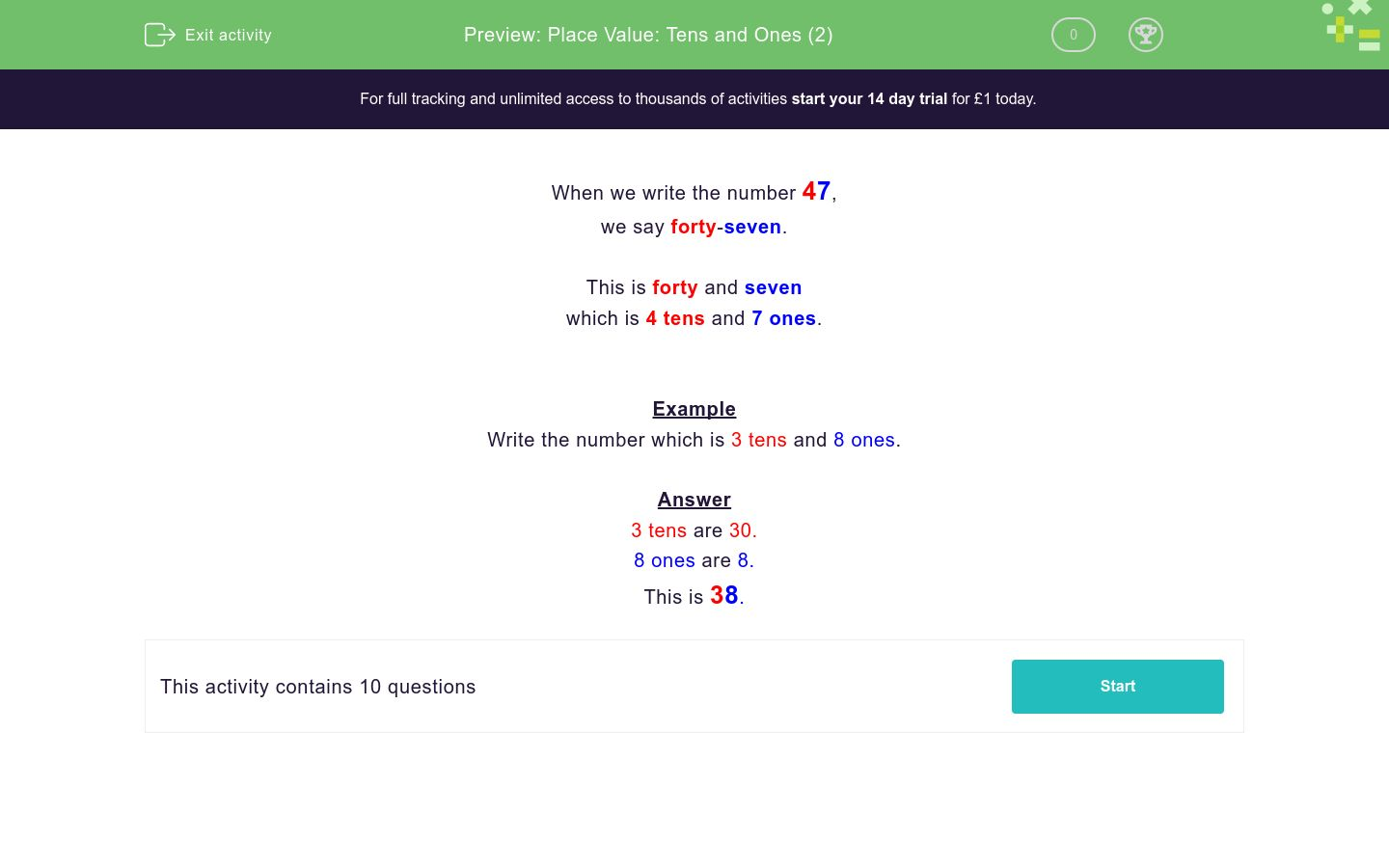# Place Value: Tens and Ones (2)

In this worksheet, students must write a number given in tens and ones as a proper number.Key stage:  KS 1

Curriculum topic:   Number: Number and Place Value

Curriculum subtopic:   Recognise Tens and Ones

Difficulty level:### QUESTION 1 of 10

When we write the number 47,

we say forty-seven.

This is forty and seven

which is 4 tens and 7 ones.

Example

Write the number which is 3 tens and 8 ones.

3 tens are 30.

8 ones are 8.

This is 38.

Write the number which is:

3 tens and 7 ones

Write the number which is:

5 tens and 7 ones

Write the number which is:

4 tens and 8 ones

Write the number which is:

6 tens and 2 ones

Write the number which is:

1 ten and 2 ones

Write the number which is:

3 tens and 9 ones

Write the number which is:

9 tens and 7 ones

Write the number which is:

7 tens and 9 ones

Write the number which is:

1 ten and 6 ones

Write the number which is:

5 tens and 5 ones

• Question 1

Write the number which is:

3 tens and 7 ones

37
EDDIE SAYS
thirty and seven
• Question 2

Write the number which is:

5 tens and 7 ones

57
EDDIE SAYS
fifty and seven
• Question 3

Write the number which is:

4 tens and 8 ones

48
EDDIE SAYS
forty and eight
• Question 4

Write the number which is:

6 tens and 2 ones

62
EDDIE SAYS
sixty and two
• Question 5

Write the number which is:

1 ten and 2 ones

12
EDDIE SAYS
ten and two
• Question 6

Write the number which is:

3 tens and 9 ones

39
EDDIE SAYS
thirty and nine
• Question 7

Write the number which is:

9 tens and 7 ones

97
EDDIE SAYS
ninety and seven
• Question 8

Write the number which is:

7 tens and 9 ones

79
EDDIE SAYS
seventy and nine
• Question 9

Write the number which is:

1 ten and 6 ones

16
EDDIE SAYS
ten and six
• Question 10

Write the number which is:

5 tens and 5 ones

55
EDDIE SAYS
fifty and five
---- OR ----